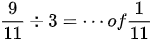# Cambridge Primary Checkpoint 2015 - Test 1

4/6/2021 2:27:00 PM

Add P to the number 8, the result is divisible by 14. P could be the following EXCEPT

• 6
• 8
• 20
• 34

104 – 48 : 8 × 2 + 4 = _____ + 16

What is the missing number?

• 2
• 68
• 78
• 80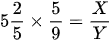If X is 4 more than Y, find the value of Y.

•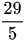•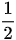• 2
•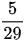The length of the base of the triangle is 1/4 of its height. Find the area of the triangle.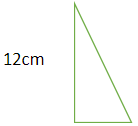• 18 cm2

• 36 cm2

• 108 cm2

• 512 cm2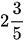h plus 48 minutes equals _____ h.

•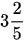•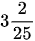•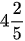•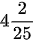A pillow costs 5 times as much as three towels. The total cost of a pillow and a towel is \$40. Find the cost of a dozen towels: _____.

• 10
• 15
• 20
• 30

Which of the following is smaller than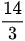and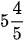?

•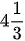• 4.7
•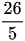•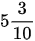The figure below shows a shaded triangle in a square. Find the area of the triangle.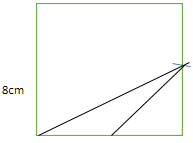• 8 cm2

• 16 cm2

• 32 cm2

• 48 cm2

Round off 451602 ÷ 100 to the nearest tens.

• 4510
• 4520
• 45160
• 45200

Kenneth faces Southeast after making a 135⁰ anticlockwise turn. Where would he be facing if he makes a 45⁰ clockwise turn instead?

• Northwest
• Northeast
• Southwest
• West

In the figure, PQ and RS are straight lines. Find the value of ∠a - ∠b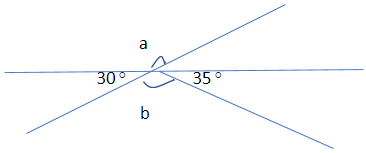• 5⁰

• 25⁰

• 30⁰

• 35⁰

A blouse costs as much as 6/5 of a shirt. A blouse and two shirts cost \$112. How much more does a blouse cost than a shirt?

• \$7
• \$22.40
• \$35
• \$42

What must be subtracted from 728054 to make 3/5 million?

In 692178, subtract the value of its smallest digit from the value of its largest digit.

Estimate the product of 798 × 992. How many ten thousands are there in the result?

In a car park, there are 135 cars and some motorcycles. If 1/6 of the vehicles are motorcycles, how many wheels are there in the car park?

The figure below shows a rectangle, ABCD, and a triangle, BDF. The length of BE to the length of BC is in the ratio 1 : 3 ABF is a straight line and AB is 16 cm longer than BF. Given that the length of BF = 20 cm. Find the total area of the figure.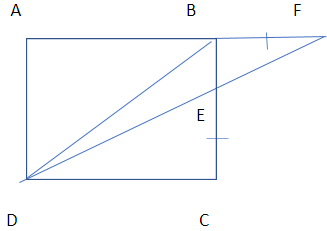What is the missing number in the box?